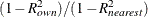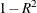# The VARCLUS Procedure

## Getting Started: VARCLUS Procedure

This example demonstrates how you can use PROC VARCLUS to cluster variables.

The following data are job ratings of police officers. The officers were rated by their supervisors on 13 job skills on a scale from 1 to 9. There is also an overall rating that is not used in this analysis. The following DATA step creates the SAS data set `JobRat`:

```data JobRat;
input
(Communication_Skills
Problem_Solving
Learning_Ability
Judgement_under_Pressure
Observational_Skills
Willingness_to_Confront_Problems
Interest_in_People
Interpersonal_Sensitivity
Desire_for_Self_Improvement
Appearance
Dependability
Physical_Ability
Integrity
Overall_Rating)
(1.);
datalines;
26838853879867
74758876857667
56757863775875
67869777988997
99997798878888

... more lines ...

99997899799799
99899899899899
76656399567486
;
```

The following statements cluster the variables:

```proc varclus data=JobRat maxclusters=3;
var Communication_Skills--Integrity;
run;
```

The DATA= option specifies the SAS data set `JobRat` as input.

The MAXCLUSTERS=3 option specifies that no more than three clusters be computed. By default, PROC VARCLUS splits and optimizes clusters until all clusters have a second eigenvalue less than one. In this example, the default setting would produce only two clusters, but going to three clusters produces a more interesting result.

The VAR statement lists the numeric variables (`Communication_Skills` `-``-` `Integrity`) to be used in the analysis. The overall rating is omitted from the list of variables.

Although PROC VARCLUS displays output for one cluster, two clusters, and three clusters, the following figures display only the final analysis for three clusters.

For each cluster, Figure 120.1 displays the number of variables in the cluster, the cluster variation, the total explained variation, and the proportion of the total variance explained by the variables in the cluster. The variance explained by the variables in a cluster is similar to the variance explained by a factor in common factor analysis, but it includes contributions only from the variables in the cluster rather than from all variables.

The line labeled "Total variation explained" in Figure 120.1 gives the sum of the explained variation over all clusters. The final "Proportion" represents the total explained variation divided by the sum of cluster variation. This value, 0.6715, indicates that about 67% of the total variation in the data can be accounted for by the three cluster components.

Figure 120.1: Cluster Summary for Three Clusters from PROC VARCLUS

Oblique Principal Component Cluster Analysis

Cluster Summary for 3 Clusters
Cluster Members Cluster
Variation
Variation
Explained
Proportion
Explained
Second
Eigenvalue
1 6 6 3.771349 0.6286 0.7093
2 5 5 3.575933 0.7152 0.5035
3 2 2 1.382005 0.6910 0.6180

 Total variation explained = 8.729286 Proportion = 0.6715

Figure 120.2 shows how the variables are clustered. Figure 120.2 also displays the R-square value of each variable with its own cluster and the R-square value with its nearest cluster. The R-square value for a variable with the nearest cluster should be low if the clusters are well separated. The last column displays the ratio offor each variable. Small values of this ratio indicate good clustering.

Figure 120.2: R-Square Values from PROC VARCLUS

3 Clusters R-squared with 1-R**2
Ratio
Cluster Variable Own
Cluster
Next
Closest
Cluster 1 Communication_Skills 0.6403 0.3599 0.5620
Problem_Solving 0.5412 0.2895 0.6458
Learning_Ability 0.6561 0.1692 0.4139
Observational_Skills 0.6889 0.2584 0.4194
Willingness_to_Confront_Problems 0.6480 0.3402 0.5335
Desire_for_Self_Improvement 0.5968 0.3473 0.6177
Cluster 2 Judgement_under_Pressure 0.6263 0.3719 0.5950
Interest_in_People 0.8122 0.1885 0.2314
Interpersonal_Sensitivity 0.7566 0.1387 0.2826
Dependability 0.6163 0.4419 0.6875
Integrity 0.7645 0.2724 0.3237
Cluster 3 Appearance 0.6910 0.3047 0.4444
Physical_Ability 0.6910 0.1871 0.3801

Figure 120.3 displays the standardized scoring coefficients that are used to compute the first principal component of each cluster. Since each variable is assigned to one and only one cluster, each row of the scoring coefficients contains only one nonzero value.

Figure 120.3: Standardized Scoring Coefficients from PROC VARCLUS

Standardized Scoring Coefficients
Cluster 1 2 3
Communication_Skills 0.212170 0.000000 0.000000
Problem_Solving 0.195058 0.000000 0.000000
Learning_Ability 0.214781 0.000000 0.000000
Judgement_under_Pressure 0.000000 0.221313 0.000000
Observational_Skills 0.220086 0.000000 0.000000
Willingness_to_Confront_Problems 0.213452 0.000000 0.000000
Interest_in_People 0.000000 0.252025 0.000000
Interpersonal_Sensitivity 0.000000 0.243245 0.000000
Desire_for_Self_Improvement 0.204848 0.000000 0.000000
Appearance 0.000000 0.000000 0.601493
Dependability 0.000000 0.219544 0.000000
Physical_Ability 0.000000 0.000000 0.601493
Integrity 0.000000 0.244507 0.000000

Figure 120.4 displays the cluster structure and the intercluster correlations. The structure table displays the correlation of each variable with each cluster component. The table of intercorrelations contains the correlations between the cluster components.

Figure 120.4: Cluster Correlations and Intercorrelations from PROC VARCLUS

Cluster Structure
Cluster 1 2 3
Communication_Skills 0.800169 0.599909 0.427341
Problem_Solving 0.735630 0.538017 0.425463
Learning_Ability 0.810014 0.411316 0.376333
Judgement_under_Pressure 0.609876 0.791401 0.345399
Observational_Skills 0.830021 0.407807 0.508305
Willingness_to_Confront_Problems 0.805002 0.362927 0.583265
Interest_in_People 0.434138 0.901225 0.387770
Interpersonal_Sensitivity 0.372371 0.869826 0.287658
Desire_for_Self_Improvement 0.772554 0.589334 0.494842
Appearance 0.552003 0.393759 0.831266
Dependability 0.664778 0.785073 0.574460
Physical_Ability 0.432590 0.416070 0.831266
Integrity 0.521876 0.874342 0.477885

Inter-Cluster Correlations
Cluster 1 2 3
1 1.00000 0.60851 0.59223
2 0.60851 1.00000 0.48711
3 0.59223 0.48711 1.00000

PROC VARCLUS next displays the summary table of statistics for the cluster history (Figure 120.5). The first three columns give the number of clusters, the total variation explained by clusters, and the proportion of variation explained by clusters, respectively.

As displayed in the first row of Figure 120.5, the variation explained by the first principal component of all the variables is 6.547402, and the proportion of variation explained is 0.5036.

When the number of clusters is two, the total variation explained is 7.96775 and the proportion of variation explained by the two clusters is 0.6129. The larger second eigenvalue of the clusters is 0.937902; so by default, PROC VARCLUS would stop splitting clusters at this point. But because the MAXCLUSTERS=3 option was specified in this example, PROC VARCLUS continues to the three-cluster solution.

When the number of clusters increases to three, the total variation explained is 8.729286 and the proportion of variation explained by the two clusters is 0.6715. The largest second eigenvalue of the clusters is 0.709323. The statistical improvement from increasing the number of clusters from two to three seems modest, but the interpretability of the three clusters argues for the three-cluster solution.

Figure 120.5 also displays the minimum proportion of variance explained by a cluster, the minimum R square for a variable, and the maximum () ratio for a variable. The last quantity is the maximum ratio of the valuefor a variable’s own cluster to the valuefor its nearest cluster.

Figure 120.5: Final Cluster Summary Table from PROC VARCLUS

Number
of
Clusters
Total
Variation
Explained
by
Clusters
Proportion
of
Variation
Explained
by Clusters
Minimum
Proportion
Explained
by a
Cluster
Maximum
Second
Eigenvalue
in a
Cluster
Minimum
R-squared
for a
Variable
Maximum
1-R**2
Ratio
for a
Variable
1 6.547402 0.5036 0.5036 1.772715 0.2995
2 7.967753 0.6129 0.5475 0.937902 0.3123 0.8026
3 8.729286 0.6715 0.6286 0.709323 0.5412 0.6875